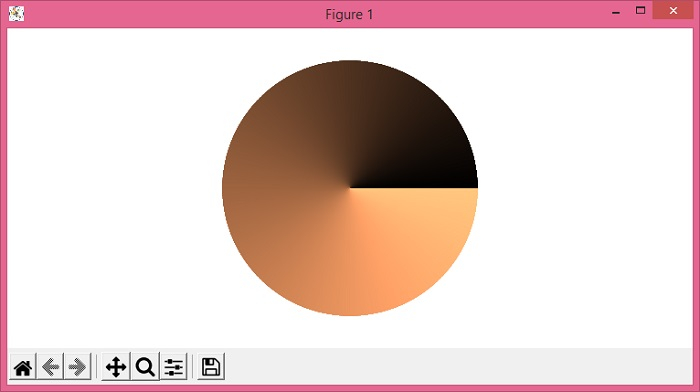# Plot a polar color wheel based on a colormap using Python/Matplotlib

To plot a color wheel based on a colormap using Python/Matplotlib, we can use the colorbar class and can use copper colormap.

## Steps

• Set the figure size and adjust the padding between and around the subplots.

• Create a new figure or activate an existing figure using figure() method.

• Set the direction of the axes.

• Linearly normalize the data using Normalize class.

• Draw a colorbar in an existing axes.

• Set the artist's visibility.

• Turn the X- and Y-axis off.

• To display the figure, use show() method.

## Example

import numpy as np
from matplotlib import pyplot as plt, cm, colors, colorbar
plt.rcParams["figure.figsize"] = [7.50, 3.50]
plt.rcParams["figure.autolayout"] = True
fig = plt.figure()
display_axes = fig.add_axes([0.1, 0.1, 0.8, 0.8], projection='polar')
display_axes._direction = 2 * np.pi
norm = colors.Normalize(0.0, 2 * np.pi)
cb = colorbar.ColorbarBase(display_axes, cmap=cm.get_cmap('copper', 2056), norm=norm, orientation='horizontal')
cb.outline.set_visible(False)
display_axes.set_axis_off()
plt.show()

## Output